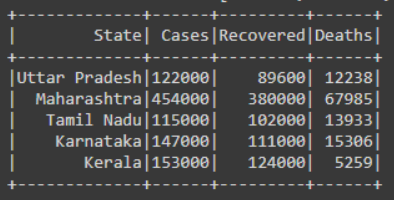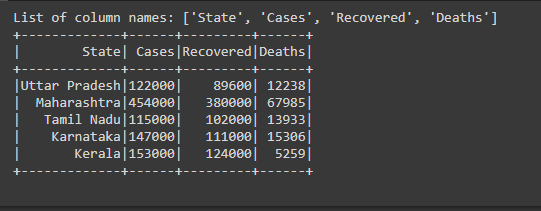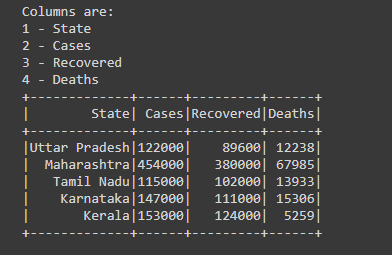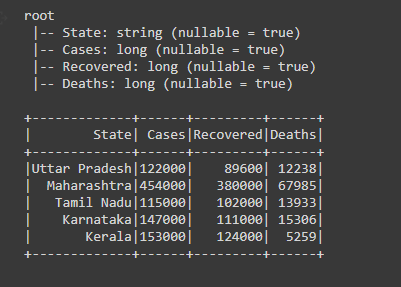Open In App

# How to get name of dataframe column in PySpark ?

In this article, we will discuss how to get the name of the Dataframe column in PySpark.

To get the name of the columns present in the Dataframe we are using the columns function through this function we will get the list of all the column names present in the Dataframe.

Syntax:

`df.columns`

We can also get the names of the columns from the list of StructFields then extract the name of the columns from the list of StructFields.

Syntax:

`df.schema.fields`

Let’s create a sample dataframe given below:

## Python

 `# importing necessary libraries``from` `pyspark.sql ``import` `SparkSession`  `# function to create new SparkSession``def` `create_session():``    ``spk ``=` `SparkSession.builder \``        ``.master(``"local"``) \``        ``.appName(``"Product_details.com"``) \``        ``.getOrCreate()``    ``return` `spk` `def` `create_df(spark, data, schema):``    ``df1 ``=` `spark.createDataFrame(data, schema)``    ``return` `df1`  `if` `__name__ ``=``=` `"__main__"``:` `    ``input_data ``=` `[(``"Uttar Pradesh"``, ``122000``, ``89600``, ``12238``),``                  ``(``"Maharashtra"``, ``454000``, ``380000``, ``67985``),``                  ``(``"Tamil Nadu"``, ``115000``, ``102000``, ``13933``),``                  ``(``"Karnataka"``, ``147000``, ``111000``, ``15306``),``                  ``(``"Kerala"``, ``153000``, ``124000``, ``5259``)]` `    ``# calling function to create SparkSession``    ``spark ``=` `create_session()` `    ``schema ``=` `[``"State"``, ``"Cases"``, ``"Recovered"``, ``"Deaths"``]` `    ``# calling function to create dataframe``    ``df ``=` `create_df(spark, input_data, schema)` `    ``# visualizing the dataframe``    ``df.show()`

Output:Example 1: Using df.columns

In the example, we have created the Dataframe, then we’re getting the list of column names present in the Dataframe using df.columns then we have printed the list of column names.

## Python

 `# getting the list of column names``col ``=` `df.columns` `# printing``print``(f``'List of column names: {col}'``)` `# visualizing the dataframe``df.show()`

Output:Example 2: Using df.schema.fields

In the example, we have created the Dataframe, then we are getting the list of StructFields that contains the name of the column, datatype of the column, and nullable flag.

We have stored this list of StructFields in the variable named as ‘field’ then iterate the for loop of field and for getting the count of iteration we have taken the count of and used enumerate() function for getting the count from 1 onwards we have passed 1 after passing the field in the enumerate() function. Then print the count and names of the column simultaneously.

## Python

 `# getting the list of StructFields``field ``=` `df.schema.fields` `# using for loop to iterate and enumerate``# for indexing or numbering``for` `count, col_name ``in` `enumerate``(field, ``1``):``  ` `    ``# printing the column names``    ``print``(count, ``"-"``, col_name.name)` `    ``# visualizing the dataframe``    ``df.show()`

Output:Example 3: Using df.printSchema()

Another way of seeing or getting the names of the column present in the dataframe we can see the Schema of the Dataframe, this can be done by the function printSchema() this function is used to print the schema of the Dataframe from that scheme we can see all the column names.

## Python

 `# printing Dataframe schema to``# get the column names``df.printSchema()` `# visualizing the dataframe``df.show()`

Output: